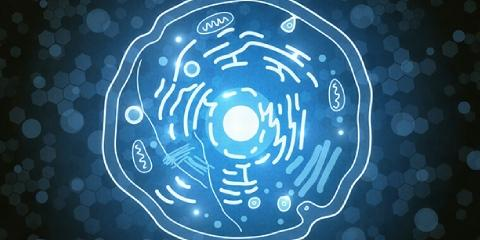# Trivia Quiz: Can You Pass This Toughest Biology Test?

70 Questions | Total Attempts: 323SettingsBiology is one of the things that give us a glimpse of life on a whole new level. Do you think that you have enough information about biology to take up what is considered the toughest biology quiz? Take up the quiz below and get to find out just how much you know — all the best as you tackle it.

• 1.
It is a method of obtaining knowledge about nature:
• A.

Science

• B.

Psychology

• C.

Cosmology

• 2.
The Scientific Method is a means of gathering information and testing ideas.  The steps to the Scientific Method are as follows:
• A.

Investigate, Experiment, Create Hypothesis, Report Discrepancy

• B.

Create Time Chart, Share Results, Chart Experience,

• C.

Observation/Question, Research Topic, Construct Hypothesis, Testing/Experimentation, Analyze Results, Draw Conclusions

• 3.
It is a possible answer to a question about nature based upon observations, research and a knowledge of the investigator.
• A.

Conclusion

• B.

Hypothesis

• C.

Metric System

• 4.
If a hypothesis is repeatedly supported by further experimentation and verified by other scientists, it may become this:
• A.

Theory

• B.

Experimental Conclusion

• C.

Determined Factor

• 5.
The word biology contains the parts:"bio"-  which means life         and        -"logy"  which means study ofMaking the definition of biology:
• A.

Life hypothesis and experimentation

• B.

Development of theories on life

• C.

The study of life

• 6.
"Micro" is a prefix that means tiny or small.  The word Microbiologist means:
• A.

Study of microorganisms, which are organisms too small to be seen with the un-aided eye.

• B.

Study of microns

• C.

Biology of animals bigger than an ant but smaller than whales

• 7.
Ornithology is the study of:
• A.

Flowers

• B.

The Ocean

• C.

Birds

• 8.
In the metric system, a unit of length is called a(n):
• A.

Mile (mi)

• B.

Meter (m)

• C.

Cosine

• 9.
Grams are the unit of measure of what, according to the Metric System:
• A.

Volume

• B.

Mass

• C.

Velocity

• 10.
The metric system measures volume with this unit:
• A.

Liter (l)

• B.

Meter

• C.

Liquid Ounce

• 11.
Mega- is the Metric System prefix that means "one million", and has a multiplier of:
• A.

.001

• B.

1,000,000

• C.

200,000

• 12.
The metric system basic unit of time is the:
• A.

Second (s)

• B.

Minute (min)

• C.

Hour (hr)

• 13.
The metric system basic unit of temperature is measured in:
• A.

Degrees Farenheit

• B.

Kelvin temperature

• C.

Degrees Celsius

• 14.
Abiogenesis is another word for this phrase, that refers to the hypothesis that living things can originate from nonliving matter.  (NOTE:  The Greek teacher Aristotle (383-322 B.C.) believed in this hypothesis.)
• A.

Natural Selection

• B.

Spontaneous Generation

• C.

Big Bang Theory

• 15.
This Italian physician and scientist who listed in the 1600's, performed experiments which disproved Aristotle's theory of Spontaneous Generation:
• A.

Francesco Redi

• B.

Leonardo DaVinci

• C.

Marcel Marceaux

• 16.
Spontaneous Generation:
• A.

Abiogenesis

• B.

Abolition

• C.

Inanimation

• 17.
Louis Pasteur's (1822-1895) work supported the hypothesis of:
• A.

Abiogenesis

• B.

Microbiology

• C.

Biogenesis

• 18.
Characteristics of living things:
• A.

Generates Energy; Breaks Down Cells; Has A Magnetic Field; Is Non-responsive to Light

• B.

Use Energy; Cellular; Grow; Metabolizes; Reproduces; Responds To Environment; Movement

• C.

Metabolize; Digest; Assimilate; Excrete Water; Anchor In One Dimension

• 19.
Anything that has mass and takes up space is this:
• A.

Matter

• B.

Volume

• C.

Traction

• 20.
Basic unit of an element:
• A.

Proton

• B.

Nucleotide

• C.

Atom

• 21.
Which group is statements are all correct?
• A.

Proton is positively charged particle; Electron is negatively charged particle; Neutron is an electrically neutral particle.

• B.

Proton is positively charged particle; Electron has only an East magnetic pole; Neutron only has a North magnetic pole.

• C.

Protein is negatively charged particle; Electron is positively charged particle; Neutron has both North and South electric poles.

• 22.
Matter that only has one type of atom is this:
• A.

Nucleus

• B.

Element

• C.

Aurum

• 23.
Hydrogen has how many protons?
• A.

1

• B.

2

• C.

3

• 24.
Carbon has these many atoms:
• A.

2

• B.

4

• C.

6

• 25.
Atomic Number is equal to the number of Protons.
• A.

True

• B.

False

• C.

Sometimes

Related TopicsBack to top